# Consequences Of Jahn-Teller Distortion - Coordination Chemistry Notes | Study Inorganic Chemistry - Chemistry

## Chemistry: Consequences Of Jahn-Teller Distortion - Coordination Chemistry Notes | Study Inorganic Chemistry - Chemistry

The document Consequences Of Jahn-Teller Distortion - Coordination Chemistry Notes | Study Inorganic Chemistry - Chemistry is a part of the Chemistry Course Inorganic Chemistry.
All you need of Chemistry at this link: Chemistry

Consequences of Jahn-Teller Distortion

• Stability of Cu2+ Complexes: For a given ligand, the relative stabilities of complexes of dipositive metal ions of first transition series is in order

Ba2+ < Sr2+ < Ca2+ < Mg2+ < Mn2+ < Fe2+ < Co2+ < Ni2+ < Cu2+ > Zn2+

This is called Irving-William Series. The extra stability of Cu2+ complexes is due to the Jahn-Teller distortion. During distortion two electrons are lowered in energy while one is raised an equal amount of energy.
• The complex [Cu(en)3]2+ is unstable because Jahn-Teller distortion causes strain into ethylenediamine molecule attached along z-axis.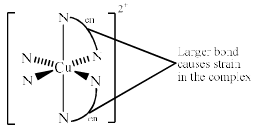Similarly, trans- [Cu(en)2(H2O)2] 2+ is more stable than the cis-[Cu(en)2(H2O)2]2+ because Jahn-Teller distortion causes strain in ethylenediamine molecule along z-axis in the later.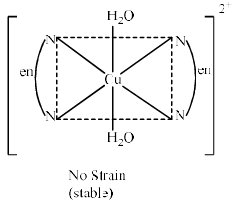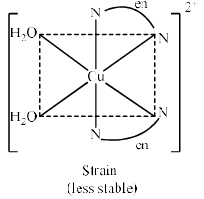•  In the cr ystalline KCuF3, the two Cu-F distances on z-axis are 196 pm and the other four Cu-F distances in xy plane are 207 pm. This is due to the Jahn-Teller distortion (compression).
• Complexes of Au2+ (d9 configuration) ion are unstable and undergo disproportionation to Au (I) and Au (III) whereas the complexes of Cu2+ (d9) Ag2+ (d9) are comparatively more stable. We know that crystal field splitting value increases on moving down the group. The high value of ∆ causes the high destabilization of dx2 – y2 orbital. Therefore, Au2+ becomes extremely reactive and undergoes oxidation to Au3+, a d8 system or reduction to Au+, a d10 system. Au3+ forms a square planar complex while Au+ forms a linear complex.

Applications of CFSE

• Ionic Radii of Transition Metal Cations: Ionic radii of dipositive or tripositive metal ions are expected to decrease linearly from Ca2+ to Zn2+ because of increasing effective nuclear charge.  But some ions show discrepancies from this regular trend of decreasing ionic radii.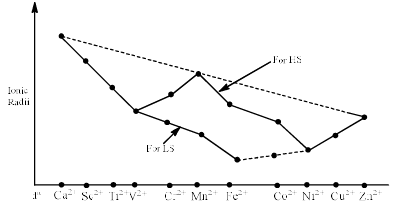The ionic radii is determined b y how closely a ligand can approach the metal ion. If both the t2g and eg orbitals are symmetrical filled the ionic radii will not be deviated. For example ionic radii of Ca2+, Mn2+ and Zn2+ fall on curve because these ions are screened by a spherical charge distribution. In d1 syst em the electron will be in t2g orbital which does not point towards ligands, therefore, the ionic radii is decreased from d1 to d3 system. When we go to d4 high spin the radii increase because the coming electron would be in eg orbital and hence ligands shall repel farther. This trend will continue and the above curve is obtained. On the basis of above statements we can also explain the trend of ionic radii obtained in the case of tripositive metal ions.

• Hydration Energy of Metal Ions: Hydration energy is the energy released when gaseous metal cations get hydrated.

Mn+(g) + 6H2O → [M(H2O)6]n+ + Hydration energy

The hydration energy of metal ions should increase linearly from Ca2+ to Zn2+ because the charge density is increase but it is not observed. This is because when the metal cations of 3d -series transition metals get hydrated, a high spin aqua complex is formed. Therefore, energy released is not only hydration energy but also CFSE. Thus:

Experimental Hydration Energy    =     Theoretical Hydration Energy      +    CFSE

If the experimental hydration energies for dipositive metal ions of the 3d transition elements are plotted as a function of atomic no., a double humped curved is formed.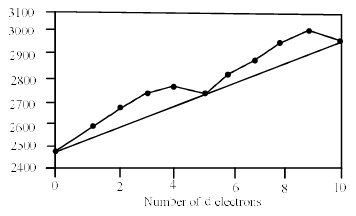The two humps in such a curve due to contribution of CFSE to the hydration energy.  Since the CFSE of Ca2+, Mn2+, and Zn2+ in high spin Oh complexes is zero. Therefore, the hydration energies of these ions lie on the straight line and does not deviate from the experimental value.

• Lattice Energy: Lattice energy is the change in energy when one mole of an ionic crystal is formed from its constituent gaseous ions.

M+ (g) + X– (g) → MX (s) + Lattice energy

According to Born Landes’s equation:

Lattice energy (U) =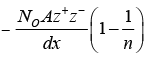i. e. Lattice energy =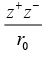Where N = Avogadro’s no., A = Madelung Constant which depends upon the geometry of the crystal, Z+ and Z are the charges on the cat ion and anion respectively, r0 = interionic distance, n = Born exponent.

According to Born Lande’s equation, the lattice energy of an ionic crystal increases with increase in product of Z+ and Z and decreases with interionic distance (r0). Consequently, the lattice energy for halides of the dipositive metal ions of the 3d -elements should increase from Ca2+ to Zn2+. But a humped curve is obtained. This is because of contribution of CFSE to the lattice energy.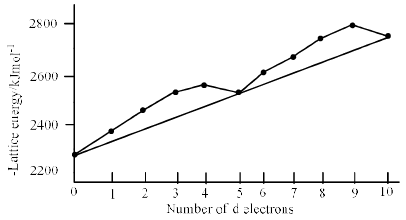• Structure of Spinels: Spinels are mixed metal oxide having formula AB2O4 or AO.B2O3 where A can be group 2 metal or a transition metal in +2 oxidation state and B can be a group 13 metal or transition metal in +3 oxidation state. The oxide anions form a cubic closed packed (ccp) or face centred cubic (fcp) lattice with four tetrahedral (Td) voids and eight octahedral (Oh) voids per AB2Ounit.

No. of tetrahedral voids = 2× no. of octahedral voids.

No. of octahedral voids = No. of lattice points.

Spinels are of two types:

(a) Normal Spinels (b) Inverse Spinels

a. Normal Spinels : In normal spinels A2+ ions occup y one eight of the tetrahedral voids and B3+ ions occupy half of the octahedral voids. This is written as [AII][BIII]O4.
For example – MgAl2O4, MgCr2O4, NiCr2O4, Mn3O4, Co3O4 etc.

b. Inverse Spinels: In inverse spinel, half of the B3+ ions have exchanged places with all the A2+ ions i.e. the A2+ occupy 1/4 of the octahedral voids along with half of the B3+ ions, whereas half of the B3+ ions occup y 1/8 of the Oh voids. It is represented as

[B][AB]O4. Examples are CrFe2O4, Fe3O4, NiFe2O4, MgFe2O4, NiAl2O4 etc. In general, the spinels having Fe3+ ions are inverse spinels.

Predicting Structures of Spinels: Structures of spinels are determined by the CFSE of the cations occupying the tetrahedral and octahedral voids.
The metal cations in Td and Oh voids are surrounded b y four and six oxide ion respectively. Thus, metal ions are considered to form Td and Oh complexes respectively. The oxide ion because as weak ligand. Structure is determined by followed way:

• Compare CFSE in Oh and Td field for both A2+ and B3+ ions
• If CFSE in Oh voids is greater than B3+ in Td and A2+ in both Td and Oh, the spinel will be normal.
• If CFSE for A2+ ions in Oh void is greater than A2+ in Td and B3+ in both Td and Oh, that will be inverse spinel.

Examples:

• Mn3O4 →MnIIMn2IIIO4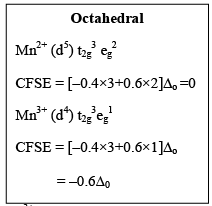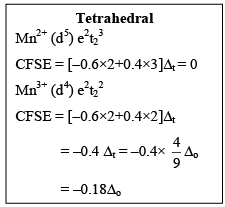Since, Mn3+ in Oh filed has the highest CFSE, therefore it will be stabilized in Oh voids. Consequently, Mn3+ will occupy the Oh voids and the Mn2+ ion will occupy the tetrahedral voids. Hence Mn3O4 is a normal spinel.

Caution: Calculate all the CFSE in terms of ∆0 for comparing. The ∆t can be converted into ∆0 by
multiplying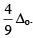. For comparing only see the magnitude and not the sign.

•  Fe3O4 → FeIIFe2IIIO4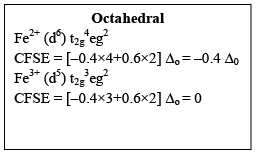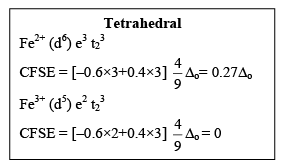Since CFSE for Fe2+ in octahedral field is highest . Therefore, Fe2+ ions will occup y Oh voids and Fe3+ ions will occupy both Td and Oh voids. Thus Fe3O4 in an inverse spinel.

• NiFe2O4 → NiIIFe2IIIO4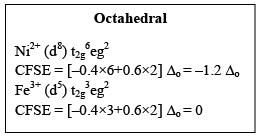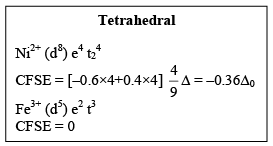Since CFSE for Ni2+ is highest in Oh field. Therefore, Ni2+ ions will occupy Oh voids and Fe3+ ions will occupy both Td and Oh voids. Thus Ni2Fe2O4 in an inverse spinel.
Note- Spinel having Fe3+ ions are inverse spinel because Fe3+ (d5) ion has CFSE equal to zero in both octahedral Td voids.

• Co3O4 → CoIICo2IIIO4
For Co3+, oxide ion behaves as strong field ligand hence low spin complex is formed.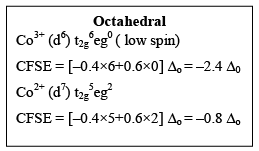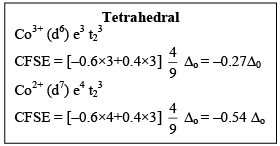Since CFSE for Co3+ is highest in Oh field, Co3+ ions will occupy octahedral and Co2+ ions will occupy tetrahedral voids. Hence Co3O4 is a normal spinel.

Shortcut → Calculate CFSE for both A2+ and B3+ ions in Oh filed. If CFSE of B3+ is higher than A2+ ions, the spinel will be normal spinel. If CFSE of A2+ is higher than that of B3+, then the spinel will be inverse spinel.

Limitations of CFT:

• Considers only d orbitals and s and p orbitals are ignored.
• Does not consider the covalent character in transition metal complexes.
• Can not explain the Spectrochemical series.
The document Consequences Of Jahn-Teller Distortion - Coordination Chemistry Notes | Study Inorganic Chemistry - Chemistry is a part of the Chemistry Course Inorganic Chemistry.
All you need of Chemistry at this link: ChemistryUse Code STAYHOME200 and get INR 200 additional OFF

## Inorganic Chemistry

49 videos|71 docs|16 tests

Track your progress, build streaks, highlight & save important lessons and more!

,

,

,

,

,

,

,

,

,

,

,

,

,

,

,

,

,

,

,

,

,

;